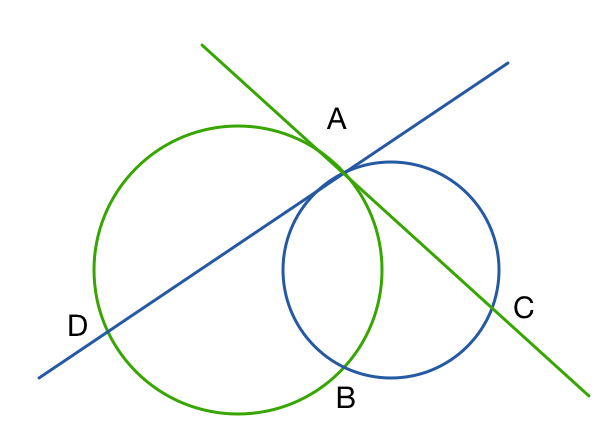# Not What It Seems2 circles intersect at $A$ and $B$.
The tangent to the first circle at $A$ intersects the second circle at $C$.
The tangent to the second circle at $A$ intersects the first circle at $D$.
If $B, C, D$ lie on a line, what can we say about $\angle DAC$?

×